# Monoid

A term used as an abbreviation for the phrase "semi-group with identity" . Thus, a monoid is a setwith an associative binary operation, usually called multiplication, in which there is an elementsuch thatfor any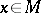. The elementis called the identity (or unit) and is usually denoted by. In any monoid there is exactly one identity. If the operation given on the monoid is commutative, it is often called addition and the identity is called the zero and is denoted by.

Examples of monoids. 1) The set of all mappings of an arbitrary setinto itself is a monoid relative to the operation of successive application (composition) of mappings. The identity mapping is the identity. 2) The set of endomorphisms of a universal algebrais a monoid relative to composition; the identity is the identity endomorphism. 3) Every group is a monoid.

Every semi-groupwithout an identity can be imbedded in a monoid. For this it suffices to take a symbolnot inand give a multiplication on the set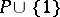as follows: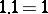,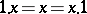for any, and on elements fromthe operation is as before. Every monoid can be represented as the monoid of all endomorphisms of some universal algebra.

An arbitrary monoid can also be considered as a category with one object. This allows one to associate with a monoidits dual (opposite, adjoint) monoid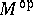. The elements of both monoids coincide, but the product ofandinis put equal to the product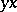in.

The development of the theory of monoids and adjoint functors has shown the utility of the definition of a monoid in so-called monoidal categories. Suppose given a category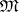equipped with a bifunctor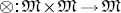, an objectand natural isomorphisms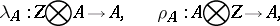satisfying coherence conditions. An objectis called a monoid in the categoryif there are morphismsand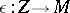such that the following diagrams are commutative: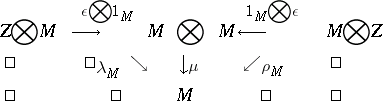Ifis taken to be the category of sets,the Cartesian product,a one-point set, and the isomorphisms,andare chosen in the natural way (,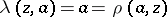), then the second definition of a monoid turns out to be equivalent to the original definition.

How to Cite This Entry:
Monoid. Encyclopedia of Mathematics. URL: http://encyclopediaofmath.org/index.php?title=Monoid&oldid=18430
This article was adapted from an original article by M.Sh. Tsalenko (originator), which appeared in Encyclopedia of Mathematics - ISBN 1402006098. See original article